SSC CGL Previous Year Question Paper 29 August 2016 - Quanti - Study24x7New to Study24X7 ?

# SSC CGL Previous Year Question Paper 29 August 2016 - Quantitative Aptitude

Updated on 15 February 2020SSC 2021 Preparation Strategie
Updated on 15 February 2020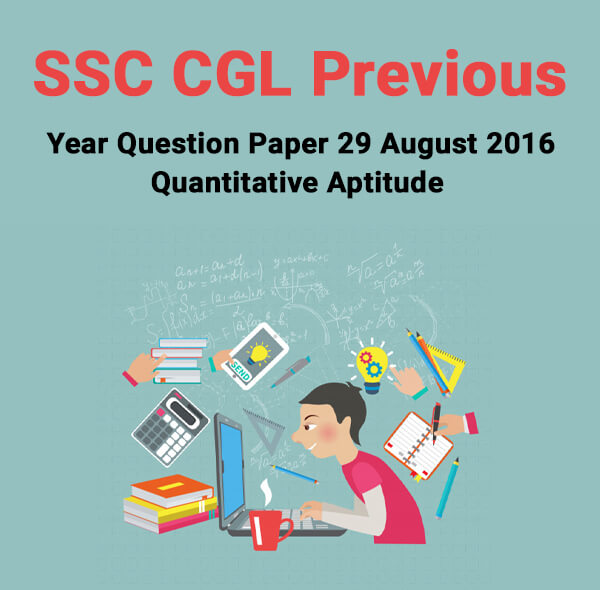Question 1. If the sum of a number and its reciprocal be 2, then the number is

a. 0

b. 1

c. -1

d. 2

Question 2.The price of a shirt after 15% discount, is Rs.119. What was the marked price of the shirt before discount

a. Rs.129

b. Rs.140

c. Rs.150

d. Rs.160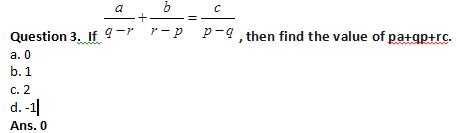Question 4.The average of a,b,c is 20 and that of b,c,d is 25; if d=30, then the value of a is

a. 25

b. 45

c. 30

d. 15

Question 5.A store sells a watch for a profit of 25% of the cost. Then the percentage of profit against selling price is

a. 22%

b. 20%

c. 18%

d. 15%

Question 6.If A is equal to 20% of B and B is equal to 25% of C; then what percent of C is equal to A?

a. 10

b. 15

c. 5

d. 20

SSC CGL tier 1 Exam : preparation strategy

Question 7.A gun is fired at a distance of 1.7 km from Ram and he hears the sound after 25 seconds. The speed of sound in meter per second is

a. 60

b. 62

c. 64

d. 68

Question 8.A sum of ₹ 3000 yields an interest of ₹ 1080 at 12% per annum simple interest in how many years ?

a. 4 Years

b. 3 Years

c. 5 years

d. 2&frac12; Years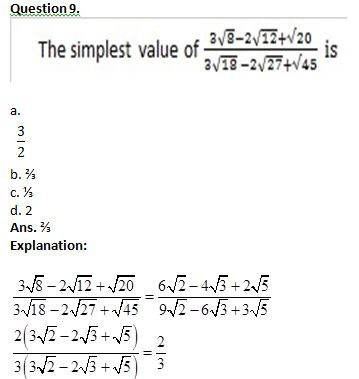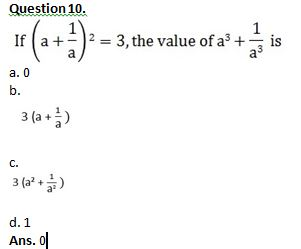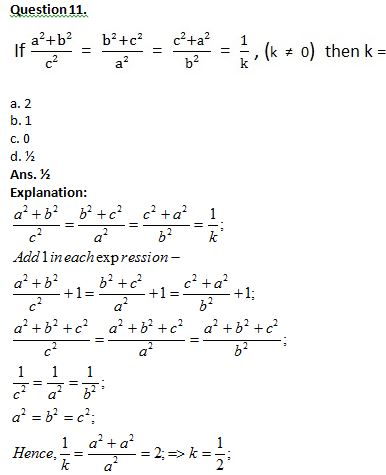Question 12.The area of the largest triangle that can be inscribed in a semicircle of radius 6m is

a. 36 m2

b. 72 m2

c. 18 m2

d. 12 m2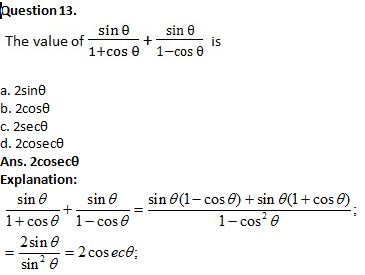Question 14.Twenty women can do a work in sixteen days. Sixteen men can complete the same work in fifteen days. The ratio between the capacity of a man and a woman is

a. 3:4

b. 4:3

c. 5:3

d. 5:7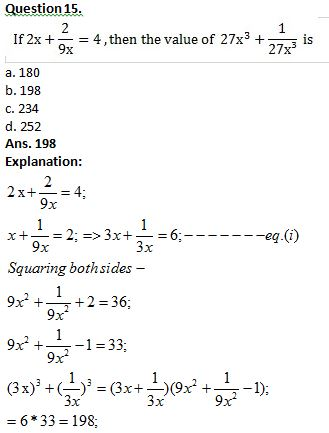Question 16.In a cyclic quadrilateral ABCD,  ∠BCD=120° and passes through the centre of the circle. Then ∠ABD = ?

a. 30°

b. 40°

c. 50°

d. 60°

Question 17.The midpoints of AB and AC of a triangle ABC are X and Y respectively. If BC+XY=12 units, then BC-XY is

a. 10 units

b. 8 units

c. 6 units

d. 4 units

Question 18.In an isosceles ΔABC, AD is the median to the unequal side meeting BC at D. DP is the angle disector of ∠ADB and PQ is drawn parallel to BC meeting AC at Q. Then the measure of ∠PDQ is

a. 130°

b. 90°

c. 180°

d. 45°

Question 19.129 meter from the foot of a cliff on level of ground, the angle of elevation of the top of a cliff is 30°. The height of this cliff is

a. 50√3 metre

b. 45√3 metre

c. 43√3 metre

d. 47√3 metre

Question 20.The volume of metallic cylindrical pipe of uniform thickness is 748 c.c. Its length is 14 cm and its external radius is 9 cm. The thickness of the pipe is

a. 0.5 cm

b. 1.5 cm

c. 1 cm

d. 2 cm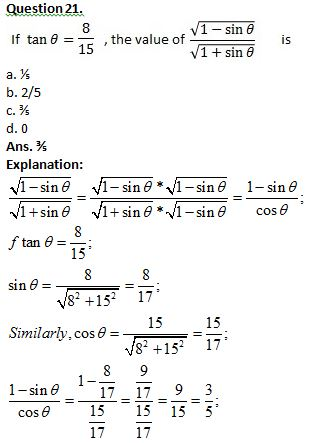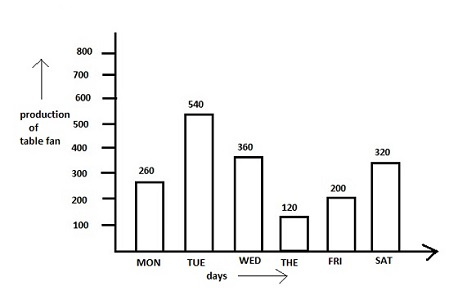Question 22. The maximum production exceeds the minimum production by:

a. 400

b. 420

c. 500

d. 540

SSC CGL 2020 Syllabus - Exam Pattern

Question 23. The average production of table fan in that week is

a. 370

b. 280

c. 300

d. 250

Question 24. Ratio of the total production of table fans in the factory from Monday to Wednesday to that from Thursday to Saturday is

a. 19:26

b. 26:19

c. 29:16

d. 16:29

Question 25. The average production of table fans on Monday & Tuesdays exceeds the average production of table fans during the week by

a. 150 fans

b. 100 fans

c. 140 fans

d. 200 fans

All The Best To All The SSC CGL 2020 Aspirants !

Write a comment...Trending ArticlesBy CBSE CLASS 8By CBSE CLASS 10By Examination Guide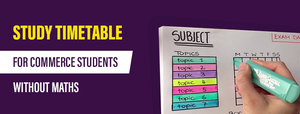By K-12-Commerce
Related Posts